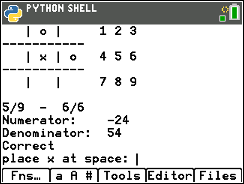### Fraction Tic Tac Toe

In this TI-84 Plus CE Python coding activity, students will create a fraction based, tic tac toe game using Python and mathematics.

•TI-84 Plus CE Python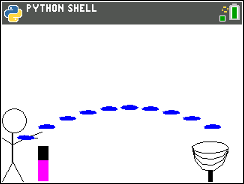### Frisbee Golf Equations

In this coding activity for the TI-84 Plus CE Python, students will create a frisbee golf game, using Python and mathematics.
•TI-84 Plus CE Python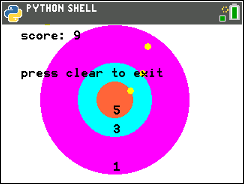### Integer Darts

In this TI-84 Plus CE Python coding activity, students will you will create an integer dart game using Python and mathematics.

•TI-84 Plus CE Python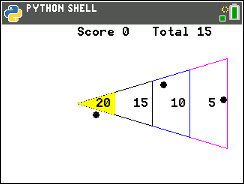### Shuffleboard Slide

In this coding activity for the TI-84 Plus CE Python, students will create a shuffleboard game using Python and mathematics.

•TI-84 Plus CE Python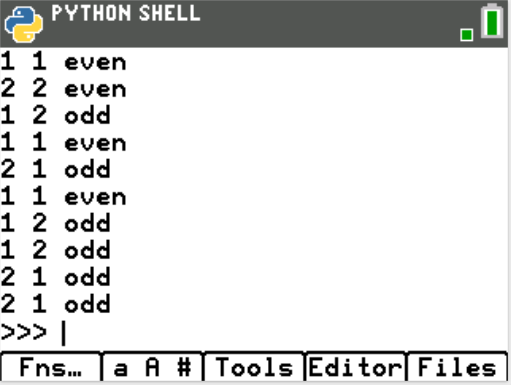### Simulating Fairness

In this coding activity for the TI-84 Plus CE Python, students will create a simulation for the game “Evens or Odds”.  The simulation will allow students to investigate the fairness of different variations of the game.

•TI-84 Plus CE Python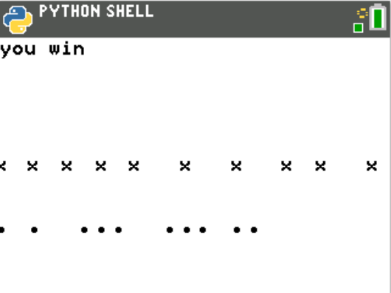In this coding activity for the TI-84 Plus CE Python, students will create a fast-paced game to help practice solving multi-step equations.

•TI-84 Plus CE Python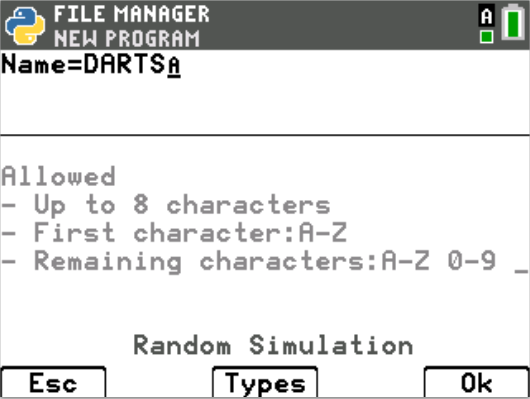### Factor Darts

In this coding activity for the TI-84 Plus CE Python, students will create a dart game that generates four random quadratic expressions. For each question the player answers correctly, the player will get another shot at the dart board.
•TI-84 Plus CE Python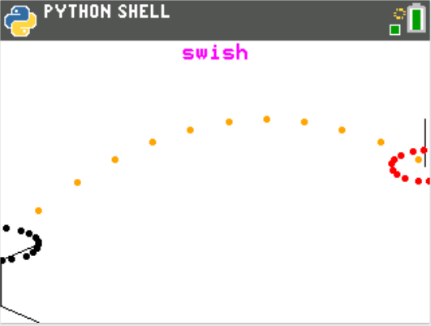### Parabolic Hoops

In this coding activity for the TI-84 Plus CE Python, students will create a virtual basketball court.  Using the location of the hoop and the vertex, the shooter will calculate the amplitude, a, needed to “Swish” nothing but net!

•TI-84 Plus CE Python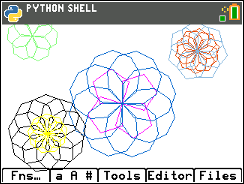### Putting the Fun in Functions

In this coding activity for the TI-84 Plus CE Python, students will use functions, translations, and rotations to create works of art.
•TI-84 Plus CE Python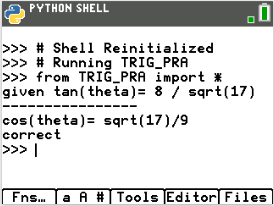### Trig Ratios: Gettin' Trig-y with Python

In this coding activity for the TI-84 Plus CE Python edition, students will write a program that generates a random sine, cosine or tangent ratio.  It will ask the user to enter a different trigonometric ratio based on the given triangle information. It will evaluate the user’s input.
•TI-84 Plus CE Python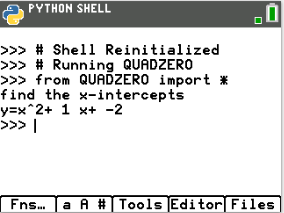In this coding activity for the TI-84 Plus CE Python edition, students will explore factored equations, and create a game to practice finding integer x-intercepts for equations.
•TI-84 Plus CE Python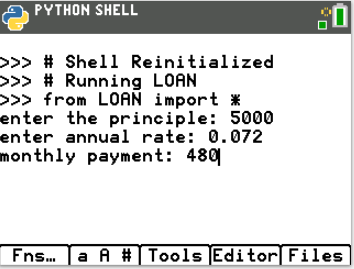### Paying off a Loan

In this coding activity for the TI-84 Plus CE Python edition, students will write a program that asks for the principle, annual interest rate and monthly payment.  The output will display the approximate number of months it will take to pay off as well as the total amount paid.
•TI-84 Plus CE Python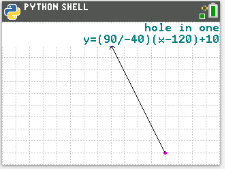### Putt Putt with Python

In this coding activity for the TI-84 Plus CE Python edition, students will create a golf game.  The golf game gives the user two random points, one for the golf ball and one for the golf hole.  The user will enter a value for the slope.
•TI-84 Plus CE Python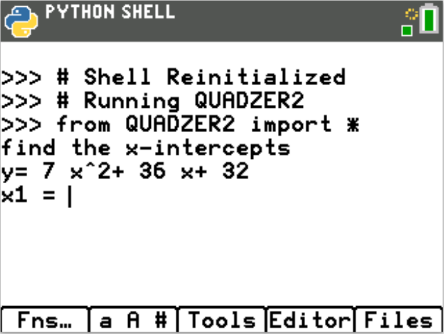••